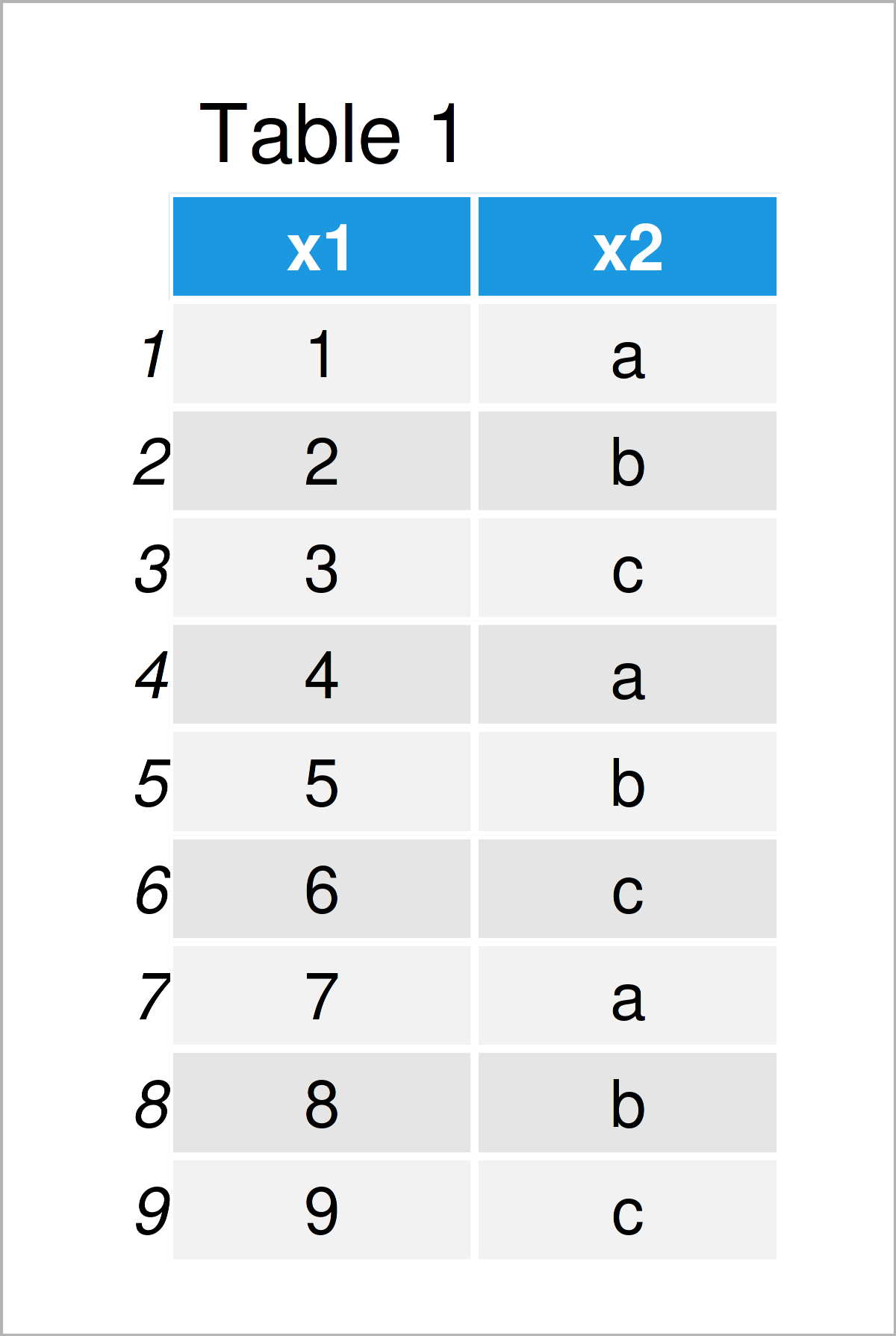# Lookup Table in R (Example)

In this R programming tutorial you’ll learn how to make a lookup table.

It’s time to dive into the exemplifying R code:

## Creation of Exemplifying Data

The first step is to create some data that we can use in the examples later on:

```data <- data.frame(x1 = 1:9,               # Create example data
x2 = letters[1:3])
data                                       # Print example data```Have a look at the table that has been returned after running the previous R programming code. It shows that our example data is made up of nine rows and two columns. The variable x1 is an integer and the variable x2 is a character.

Next, we have to create an exemplifying vector object based on which we can look up certain values in our data frame:

```vec <- letters[1:2]                        # Create example vector
vec                                        # Print example vector
#  "a" "b"```

Our example vector contains the character letters a and b.

## Example: Create Lookup Table Using %in% Operator

This example demonstrates how to create a lookup table, i.e. a data frame subset based on a logical condition.

For this, we can use the %in% operator as shown below:

```data_lookup <- data[data\$x2 %in% vec, ]    # Extract matching rows
data_lookup                                # Print lookup table```Table 2 shows the output of the previous code – A subset of our data set.

## Video & Further Resources

Would you like to learn more about the creation of a lookup table? Then I recommend having a look at the following video tutorial on my YouTube channel. In the video, I explain the R programming syntax of this article in RStudio.

Note that there are many different ways to create a lookup table. In this case, we have extracted a data frame subset using a logical condition based on the values in a vector. You may modify the given code based on your specific needs.

You could have a look at the related articles on my website. Some articles are shown below:

In summary: At this point you should have learned how to create a lookup table in R. In case you have any further questions, don’t hesitate to let me know in the comments below.

Subscribe to the Statistics Globe Newsletter